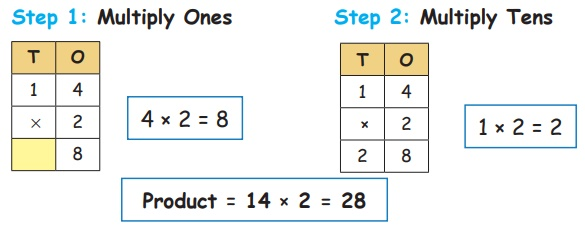Home | | Maths 3rd Std | Multiplication using standard algorithm

# Multiplication using standard algorithm

Multiply using multiplication table

Mutiplication of a number with other number can be done in the following ways.

(i) Dot multiplication

(iii) Regrouping

(iv) Standard multiplication algorithm

(v) Lattice multiplication

Multiplication using standard algorithm:

Multiply using multiplication tableProduct = 14 × 2 = 28

Example

1. Multiply. 23 × 4Product = 23 × 4 = 92

2. Multiply. 32 × 5Product = 32 × 5 = 160

Tags : Numbers | Term 2 Chapter 1 | 3rd Maths , 3rd Maths : Term 2 Unit 1 : Numbers
Study Material, Lecturing Notes, Assignment, Reference, Wiki description explanation, brief detail
3rd Maths : Term 2 Unit 1 : Numbers : Multiplication using standard algorithm | Numbers | Term 2 Chapter 1 | 3rd Maths